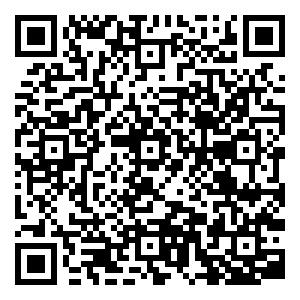2.793

2018影响因子

(CJCR)

• 中文核心
• EI
• 中国科技核心
• Scopus
• CSCD
• 英国科学文摘

## 留言板引用本文: 姚红革, 张玮, 杨浩琪, 喻钧. 深度强化学习联合回归目标定位. 自动化学报, 2020, 41(x): 1−10Yao Hong-Ge, Zhang Wei, Yang Hao-Qi, Yu Jun. Joint regression object localization based on deep reinforcement learning. Acta Automatica Sinica, 2020, 41(x): 1−10 doi: 10.16383/j.aas.c200045
 Citation: Yao Hong-Ge, Zhang Wei, Yang Hao-Qi, Yu Jun. Joint regression object localization based on deep reinforcement learning. Acta Automatica Sinica, 2020, 41(x): 1−10## Joint Regression Object Localization Based on Deep Reinforcement Learning

• 摘要: 为了模拟人眼的视觉注意机制, 快速、高效地搜索和定位图像目标, 本文提出了一种基于循环神经网络的联合回归深度强化学习目标定位模型, 该模型将历史观测信息与当前时刻的观测信息融合并做出综合分析, 以训练Agent(智能体)快速定位目标, 并联合回归器对Agent所定位的目标包围框进行精细调整.实验表明, 所提出的模型能够在少数时间步内快速、准确地定位目标.
• 图  1  状态信息融合表示

Fig.  1  Fusion representation of state information

图  2  动作示意图

Fig.  2  Schematic diagram of action

图  3  模型整体结构图

Fig.  3  Overall structure of the model

图  4  融合网络 ${f}_{c}\left({\theta }_{c}\right)$Fig.  4  Integration network ${f}_{c}\left({\theta }_{c}\right)$图  5  动作网络 ${f}_{a}\left({\theta }_{a}\right)$Fig.  5  Action network ${f}_{a}\left({\theta }_{a}\right)$图  6  位置网络 ${f}_{l}\left({\theta }_{l}\right)$Fig.  6  Location network ${f}_{l}\left({\theta }_{l}\right)$图  7  回归网络 ${f}_{g}\left({\theta }_{g}\right)$Fig.  7  Regression network ${f}_{g}\left({\theta }_{g}\right)$图  8  动作网络训练图

Fig.  8  Action network training chart

图  10  位置网络训练图

Fig.  10  Location network training chart

图  9  回归网络训练图

Fig.  9  Regression network training chart

图  11  模型训练损失曲线图

Fig.  11  Model training loss diagram

图  12  测试结果示例一

Fig.  12  Test result example 1

图  15  测试结果示例四

Fig.  15  Test result example 4

图  16  测试结果示例五

Fig.  16  Test result example 5

图  17  测试结果示例六

Fig.  17  Test result example 6

图  13  测试结果示例二

Fig.  13  Test result example 2

图  14  测试结果示例三

Fig.  14  Test result example 3

图  18  测试结果示例IoU变化趋势示意图

Fig.  18  Schematic diagram of variation trend of IOU test result

图  19  回归器精调后IoU交叠区域示意图

Fig.  19  Schematic diagram of IOU overlapping area after fine adjustment of regressor

表  1  不同算法在VOC 2007测试集上的定位精度表现(节选部分种类)

Table  1  Positioning accuracy performance of different algorithms on VOC 2007 test set (category of excerpts)

 种类 算法 aero bike bird boat bottle bus car cat mAP Faster R-CNN 86.5 81.6 77.2 58.0 51.0 78.6 76.6 93.2 75.3 Caicedo 57.9 56.7 38.4 33.0 17.5 51.1 52.7 53.0 45.0 Bueno 56.1 52.0 42.2 38.4 22.1 46.7 42.2 52.6 44.0 UR-DRQN 59.4 58.7 44.6 36.1 28.3 55.3 48.4 52.4 47.9

表  2  不同算法平均每个epoch的定位耗时

Table  2  The average location time of each epoch in different algorithms

 算法 Faster R-CNN Caicedo Bueno UR-DRQN 定位耗时(s/epoch) 372 271 251 219
•点击查看大图
##### 计量
• 文章访问数:  37
• HTML全文浏览量:  21
• 被引次数: 0
##### 出版历程
• 收稿日期:  2020-01-20
• 录用日期:  2020-09-07

### 目录/下载:  全尺寸图片 幻灯片
• 分享
• 用微信扫码二维码

分享至好友和朋友圈